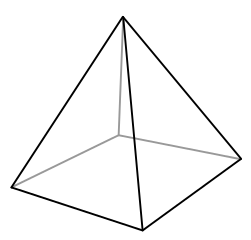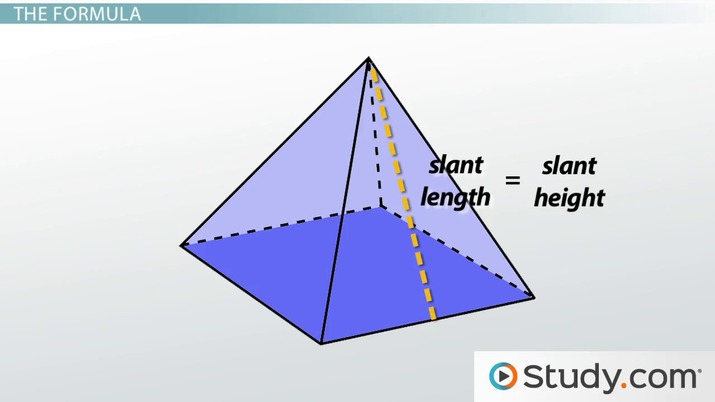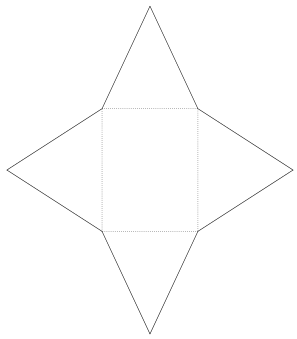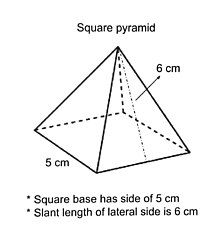# Surface Area of a Pyramid

Brigette Banaszak, Yuanxin (Amy) Yang Alcocer
• Author
Brigette Banaszak

Brigette has a BS in Elementary Education and an MS in Gifted and Talented Education, both from the University of Wisconsin. She has taught math in both elementary and middle school, and is certified to teach grades K-8.

• Instructor
Yuanxin (Amy) Yang Alcocer

Amy has a master's degree in secondary education and has taught math at a public charter high school.

What is the surface area of a pyramid? Learn the definitions of pyramid and surface area, and see how to find the surface area of a pyramid with examples. Updated: 09/11/2021

Show

## Pyramid

A pyramid is a three-dimensional solid with a flat polygon base and triangular lateral sides that come to a point.The base of a regular pyramid is a regular polygon.

Pyramids are often named by the shape of their base.

Shape of the base of pyramid Name of the pyramid
triangle triangular pyramid
square square pyramid
pentagon pentagonal pyramid
hexagon hexagonal pyramid
octagon octagonal pyramid

If a pyramid is a regular pyramid, the triangular lateral sides will be congruent, or identical in size and shape.An error occurred trying to load this video.

Try refreshing the page, or contact customer support.

Coming up next: Combined Figures: Perimeter, Area, and Volume

### You're on a roll. Keep up the good work!

Replay
Your next lesson will play in 10 seconds
• 0:01 A Pyramid
• 0:46 Surface Area
• 1:39 The Formula
• 2:35 Using the Formula
• 4:09 Lesson Summary
Save Save

Want to watch this again later?

Timeline
Autoplay
Autoplay
Speed Speed

## What Is the Surface Area of a Pyramid?

A surface area of a pyramid is the product of the area outside of the pyramid, and it is measured in square units.

The surface area of a three-dimensional polygon, called a polyhedron, can be found by finding the sum of the areas of each of the polyhedron's flat faces.

• For a regular triangular pyramid, the surface area is calculated by taking the sum of the base and the three congruent triangular sides.
• For a square pyramid, such as the one pictured here, the surface area is calculated by finding the sum of the square base and the four congruent triangular sides.• For a rectangular pyramid, the surface area is calculated by finding the sum of the rectangular base and the four triangular sides.
• For a regular pentagonal pyramid, the surface area is calculated by taking the sum of the pentagonal base and the five congruent triangular sides.
• For a regular hexagonal pyramid, the surface area is calculated by finding the sum of the hexagonal base and the six congruent triangular sides.

## How to Find the Surface Area of a Pyramid

There is a surface area of a pyramid formula. Here is how it is derived. Take a look at the square pyramid pictured here.#### Area of base

The base of the pyramid is a square. Each side of the square, s, is 5 cm. So, its area is s x s or 5 x 5 = 25. Call the area of the base B.

#### Lateral area

The lateral area is the sum of the areas of the lateral sides. Each lateral side is a triangle with a base of s (side of square base) and a height of l (slant length of a triangular side).

The slant length of the triangular side is not the same as the height of the pyramid. The slant length is greater than the height.

Each lateral side will have an area of 1/2 x s x l. Remember, there are four lateral sides. So, the total lateral area is 4 x 1/2 x s x l.

Notice that 4 x s is the perimeter of the base. The lateral area can be written as 1/2 x P x l, where P is the perimeter of the square base (4 x 5 = 20 in this example) and l is the slant length (6 in this example).

#### Total surface area

The total surface area in this example is

{eq}SA = B + \frac{1}{2} P l {/eq}

{eq}SA = 25+ \frac{1}{2} \times 20 \times 6 = 85\ square\ cm {/eq}

The formula can be generalized to apply to any regular pyramid:

{eq}SA = B + \frac{1}{2} P l {/eq}

where SA is the total surface area, and B is the pyramid's base area, both in square units, and P is the perimeter of the base and l is the slant length of the pyramid's triangular lateral sides, both in units.

To unlock this lesson you must be a Study.com Member.

#### How do you find the total surface area of a pyramid?

To find the total surface area of a pyramid, which is measured in square units, use this formula:

SA = B + 1/2 x P x l, where B is the area of the pyramid's base, P is the perimeter of the base, and l is the slant length of the lateral sides.

#### What is the formula for the surface area of a triangular pyramid?

The surface area of a triangular pyramid is SA = B + 1/2 x P x l, where B is the area of the pyramid's base, P is the perimeter of the base, and l is the slant length of the lateral sides.

### Register to view this lesson

Are you a student or a teacher?